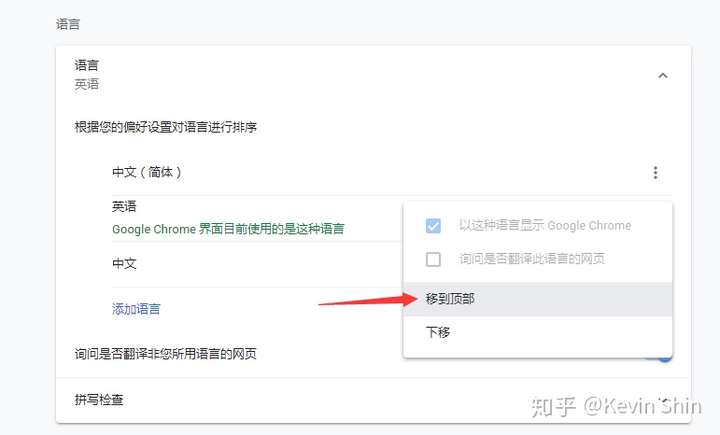# 禁止国内同行访问你的英文网站

## 一、利用 IP 地址进行判断并屏蔽

```<?php
\$verification1 = '中国'; //需要屏蔽国家名称1
\$verification2 = '摩洛哥'; //需要屏蔽的国家名称2。这里可以类似的方式，定义多个国家。
function get_visitor_ip() {
if (isset(\$_SERVER['HTTP_X_REAL_FORWARDED_FOR']) && preg_match('/^([0-9]{1,3}\.){3}[0-9]{1,3}\$/', \$_SERVER['HTTP_X_REAL_FORWARDED_FOR'])) {
\$ip = \$_SERVER['HTTP_X_REAL_FORWARDED_FOR'];
}
elseif (isset(\$_SERVER['HTTP_X_FORWARDED_FOR']) && preg_match('/^([0-9]{1,3}\.){3}[0-9]{1,3}\$/', \$_SERVER['HTTP_X_FORWARDED_FOR'])) {
\$ip = \$_SERVER['HTTP_X_FORWARDED_FOR'];
}
elseif (isset(\$_SERVER['HTTP_CLIENT_IP']) && preg_match('/^([0-9]{1,3}\.){3}[0-9]{1,3}\$/', \$_SERVER['HTTP_CLIENT_IP'])) {
\$ip = \$_SERVER['HTTP_CLIENT_IP'];
}
return \$ip;
}
\$ip = get_visitor_ip();  //获取访客IP
\$result = file_get_contents("http://ip.taobao.com/service/getIpInfo.php?ip=".\$ip);  //IP数据库来自淘宝。你也可以换成 IP138 的。建议默认。
//判断访客的IP是否来自国家1或国家2
exit();
}
?>```

## 二、利用访问者的浏览器语言类型进行判断并屏蔽

```<?php
// 定义变量 lc
\$lc = "";
// 检查是否已经设置过 HTTP头Accept-Language信息变量
if(isset(\$_SERVER['HTTP_ACCEPT_LANGUAGE']))
\$lc = substr(\$_SERVER['HTTP_ACCEPT_LANGUAGE'], 0, 2);
// 这里截取语言编码前两位来判断，如果是中文，转向百度
if(\$lc == "zh"){
exit();
}
?>```

zh中文zh-CN中文(简体)zh-HK中文(香港)zh-MO中文(澳门)zh-SG中文(新加坡)zh-TW中文(繁体)

```<?php
\$lc = '';
if(isset(\$_SERVER['HTTP_ACCEPT_LANGUAGE'])){
\$lg = \$_SERVER['HTTP_ACCEPT_LANGUAGE'];
\$lc = substr(\$_SERVER['HTTP_ACCEPT_LANGUAGE'], 0, 2);
}
\$arr = array('zh-HK','zh-TW','zh-MO','zh-SG');
if((\$lc == 'zh') && (!in_array('lg',\$arr))){
exit();
}
?>```

OK，搞定！这样就不会“误伤”其他中文语言的地区或国家的访问流量了。

## 方式二太暴力，网站站长也无法访问了，怎么办？

4.1）在网站 根目录下，放一个 php 文件。

```<html>
<title></title>
<body>
<?php
?>
</body>
</html>```

（注意：如果你使用 Avada, Betheme 等主题，如果放 <head> 上面不行，放到 `<!DOCTYPE html>` 上面即可）

```<?php
// 定义变量 lc
\$lc = "";
// 检查是否已经设置过 HTTP头Accept-Language信息变量
// 截取语言编码前两位来判断
if(isset(\$_SERVER['HTTP_ACCEPT_LANGUAGE']))
\$lg = \$_SERVER['HTTP_ACCEPT_LANGUAGE'];
\$lc = substr(\$_SERVER['HTTP_ACCEPT_LANGUAGE'], 0, 2);
\$arr = array("zh-HK","zh-TW","zh-MO","zh-SG");

if((\$lc == "zh") && (!in_array("lg",\$arr)) && (\$ck !== "authorized") ){
exit();
}
?>```

### 解决方案二：在 Chrome 设置里修改浏览器内置语言